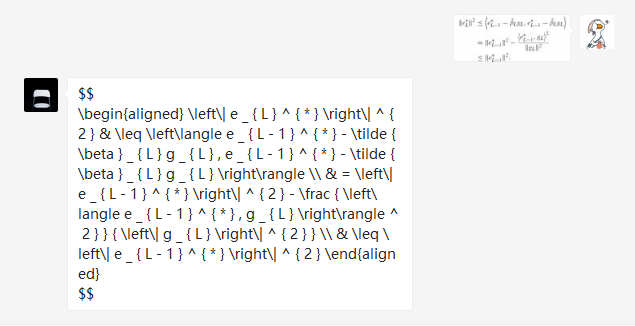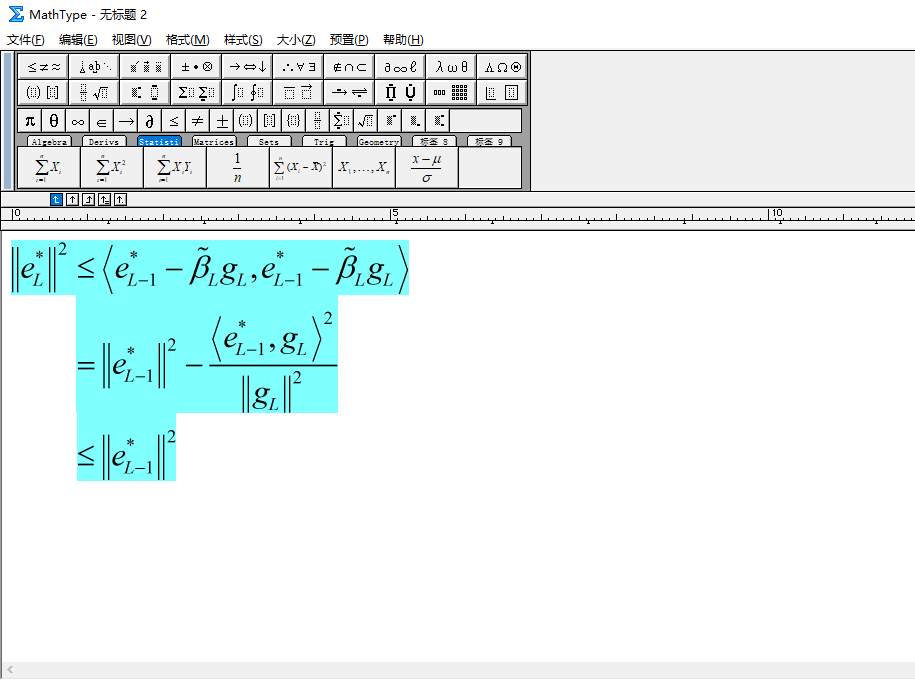• ## Math

千次阅读 2015-12-21 23:21:55
Math
java.math.Math类常用的常量和方法：

Math.PI 记录的圆周率
Math.E记录e的常量
Math.abs 求绝对值
Math.sin 正弦函数 Math.asin 反正弦函数
Math.cos 余弦函数 Math.acos 反余弦函数
Math.tan 正切函数 Math.atan 反正切函数&nbsp;Math.atan2 商的反正切函数
Math.ceil 得到不小于某数的最大整数
Math.floor 得到不大于某数的最大整数

例如：Math.floor(12.7) =12.0
Math.ceil(12.7) =13.0
ceil()是天花板，即向上取整。floor是地板，向下取整。round是四舍五入。

Math.IEEEremainder 求余
Math.max 求两数中最大
Math.min 求两数中最小
Math.sqrt 求开方
Math.pow 求某数的任意次方, 抛出ArithmeticException处理溢出异常
Math.sqrt(x)：平方根
Math.pow(x,y)：x的y次方

Math.exp 求e的任意次方
Math.log10 以10为底的对数
Math.log 自然对数
Math.rint 求距离某数最近的整数（可能比某数大，也可能比它小）
Math.round 同上，返回int型或者long型（上一个函数返回double型）
Math.random 返回0，1之间的一个随机数

java.math.BigInteger(大整数)：

BigInteger bi1=new BigInteger("1234567890123456890");
BigInteger bi2=BigInteger.valueOf(123L);
bi1=bi1.multiply(bi2);//b1*b2
bi1=bi1.subtract(bi2);//b1-b2
bi1=bi1.divide(bi2);// b1/b2
java.math.BigDecimal(大浮点数):
BigDecimal bd = new BigDecimal("3.1415926");
bd = bd.setScale(2,BigDecimal.ROUND_DOWN);//取3.1415926小数点后面二位
展开全文• ## math

千次阅读 2016-06-08 12:15:31
函数名 ...math.pi 3.1415926535898 abs 取绝对值 math.abs(-2012) 2012 ceil 向上取整 math.ceil(9.1) 10 floor 向下取整 math.floor(9.9)

函数名

描述

示例

结果

pi

圆周率

math.pi

3.1415926535898

abs

取绝对值

math.abs(-2012)

2012

ceil

向上取整

math.ceil(9.1)

10

floor

向下取整

math.floor(9.9)

9

max

取参数最大值

math.max(2,4,6,8)

8

min

取参数最小值

math.min(2,4,6,8)

2

pow

计算x的y次幂

math.pow(2,16)

65536

sqrt

开平方

math.sqrt(65536)

256

mod

取模

math.mod(65535,2)

1

modf

取整数和小数部分

math.modf(20.12)

20   0.12

randomseed

设随机数种子

math.randomseed(os.time())

random

取随机数

math.random(5,90)

5~90

角度转弧度

3.1415926535898

deg

弧度转角度

math.deg(math.pi)

180

exp

e的x次方

math.exp(4)

54.598150033144

log

计算x的自然对数

math.log(54.598150033144)

4

log10

计算10为底，x的对数

math.log10(1000)

3

frexp

将参数拆成x * (2 ^ y)的形式

math.frexp(160)

0.625    8

ldexp

计算x * (2 ^ y)

math.ldexp(0.625,8)

160

sin

正弦

0.5

cos

余弦

0.5

tan

正切

1

asin

反正弦

math.deg(math.asin(0.5))

30

acos

反余弦

math.deg(math.acos(0.5))

60

atan

反正切

math.deg(math.atan(1))

45

函数名

描述

示例

结果

pi

圆周率

math.pi

3.1415926535898

abs

取绝对值

math.abs(-2012)

2012

ceil

向上取整

math.ceil(9.1)

10

floor

向下取整

math.floor(9.9)

9

max

取参数最大值

math.max(2,4,6,8)

8

min

取参数最小值

math.min(2,4,6,8)

2

pow

计算x的y次幂

math.pow(2,16)

65536

sqrt

开平方

math.sqrt(65536)

256

mod

取模

math.mod(65535,2)

1

modf

取整数和小数部分

math.modf(20.12)

20   0.12

randomseed

设随机数种子

math.randomseed(os.time())

random

取随机数

math.random(5,90)

5~90

角度转弧度

3.1415926535898

deg

弧度转角度

math.deg(math.pi)

180

exp

e的x次方

math.exp(4)

54.598150033144

log

计算x的自然对数

math.log(54.598150033144)

4

log10

计算10为底，x的对数

math.log10(1000)

3

frexp

将参数拆成x * (2 ^ y)的形式

math.frexp(160)

0.625    8

ldexp

计算x * (2 ^ y)

math.ldexp(0.625,8)

160

sin

正弦

0.5

cos

余弦

0.5

tan

正切

1

asin

反正弦

math.deg(math.asin(0.5))

30

acos

反余弦

math.deg(math.acos(0.5))

60

atan

反正切

math.deg(math.atan(1))

45

print("floor",math.floor(1.2))
print("floor",math.floor(-1.2))
print("random",math.random())
print("random",math.random(1,10))
print("min",math.min(5,10))
结果为：
floor 1
floor -2
random  0~1直接的一个随机小数
random  1~10之间的一个随机整数

展开全文• 1.Random()、Math.random()、Math.round()函数的区别;1.Random()、Math.random()、Math.round()函数的区别;1.Random()、Math.random()、Math.round()函数的区别;1）Random()：创建一个新的随机数生成器。此构造方法...
1.Random()、Math.random()、Math.round()函数的区别;1.Random()、Math.random()、Math.round()函数的区别;1.Random()、Math.random()、Math.round()函数的区别;1）Random()：创建一个新的随机数生成器。此构造方法将随机数生成器的种子设置为某个值，该值与此构造方法的所有其他调用所用的值完全不同。	Random(long seed)使用单个long 种子创建一个新的随机数生成器。该种子是伪随机数生成器的内部状态的初始值，该生成器可通过方法 next(int) 维护。	Random 的一个特点是：相同种子数的Random对象，对应相同次数生成的随机数字是完全相同的；	Random random1 = new Random(10);	Random random2 = new Random(10);	random1、random2 对应相同次数生成的随机数字完全相同。比如：	System.out.println(ran.nextInt(10));	System.out.print(ran2.nextInt(10));	输出结果：	5	52）Math.random()能够返回带正号的double值，该值大于等于0.0且小于1.0，即取值范围是[0.0,1.0)的左闭右开区间，返回值是一个伪随机选择的数，在该范围内（近似）均匀分布。	for(int i = 0; i < 10; i++ ){		System.out.println((int)(Math.random()*5));	}	输出结果：	4，2，0，1，3，3，4，0，3，3	在使用Math.Random()的时候，需要注意的地方是该函数是返回double类型的值，所以在要赋值给其他类型的变量的时候注意需要进行塑形转换。3）（四舍五入）Math.round(float a)返回最接近参数的 int;   Math.round(double a)返回最接近参数的 long。   System.out.println(Math.round(1.5));   System.out.println(Math.round(-1.5));   System.out.println(Math.round(0.5));   System.out.println(Math.round(-0.5));   输出：	2	-1	1	0   特殊情况如下：	如果参数为 NaN，那么结果为 0。	如果结果为负无穷大或任何小于等于 Integer.MIN_VALUE 的值，那么结果等于 Integer.MIN_VALUE 的值。	如果参数为正无穷大或任何大于等于 Integer.MAX_VALUE 的值，那么结果等于 Integer.MAX_VALUE 的值。    （向上取整）Math.ceil(double a)返回最小的（最接近负无穷大） double 值，该值大于等于参数，并等于某个整数。特殊情况如下：	如果参数值已经等于某个整数，那么结果与该参数相同。	如果参数为 NaN、无穷大、正 0 或负 0，那么结果与参数相同。	如果参数值小于 0，但是大于 -1.0，那么结果为负 0。   System.out.println(Math.ceil(1.2));   System.out.println(Math.ceil(-1.2));   System.out.println(Math.ceil(0.6));   System.out.println(Math.ceil(-0.6));   输出：	2.0	-1.0	1.0	-0.0-0.0-0.0
展开全文• ## mathpix替代品终于找到了

万次阅读 热门讨论 2019-10-04 19:16:44
mathpix免费用完50次后，可以用此方法
mathpix的强大功能用的人都懂，升级后穷学生党用不起，但又不能不用的抓耳挠腮感，懂的人自然也懂。
刚知乎上发现一个公众号，同样可以将图片公式转换为letax代码形式输出，只需首次少量打赏就可以每个月使用1000次，实在是太开心了，就想分享给大家
效果如下]公众号：厦大电费
知乎号：pacoo
ps : 我只是一个小白，首次发帖，希望能造福和我一样被手敲数学公式的恐惧支配的学生狗。
再ps : 复制到mathtype之后可能需要手动调节一下公式对齐方式和删掉一些多余的代码，但这已经比手敲要简单的多了不是嘛~_~



展开全文• ## Java中Math函数的使用

万次阅读 多人点赞 2018-04-08 11:49:40
Java中Math函数的使用 说到Java中的Math函数，大家肯定不陌生，但是在真正使用的时候却犯了难，那么多方法，我们到底需要使用哪个呢？ 为此，我特地研究了一些Math常用函数的使用，以方便大家使用。 算术计算 ...
• int b=(int)(Math.random()*10);//生成[0,9]之间的随机整数。 生成从n到m的随机整数 int temp=m+(int)(Math.random()*(n+1-m)); //生成从m到n的随机整数[m,n] 原文：java Math.random()生成从n到m的随机整数 ...Java随机数
• ## Python math库

千次阅读 2018-11-22 11:50:51
import math #导包 方法 math.e #自然常数e=2.718281828459045 math.pi # 圆周率pi 3.141592653589793 math.exp(x) #返回e的x次方 math.log(x[, base]) # 返回x的以base为底的对数，base默认为e math.log10(x) #返回...
• ## math.pow()函数用法

万次阅读 2018-07-15 23:22:56
Math.pow(底数,几次方) 如：int a=3; int b=3; int c=Math.pow(a,b); 就是3的三次方是多少； c最终为27； 基础用法:用math.pow()实现数组的交错求和 int ant=0; a+=b[i]*math.pow(-1,ant); //实...java入门
• java向上取整函数Math.ceil() 百度Math.ceil()函数：“Math.ceil()是常见编程语言中的常用代码,ceil() 方法执行的是向上取整计算，它返回的是大于或等于函数参数，并且与之最接近的整数。“ Math.ceil(param)...向上取整 整除
• 1、Math.round() “四舍五入”，该函数返回的是一个四舍五入后的的整数 double d = 3.1415926; double d2 = 18.58; double d3 = -15.23; double d4 = -16.85; long round1 = Math.round(d); //...round()
• 参考https://developer.mozilla.org/zh-CN/docs/Web/JavaScript/Reference/Global_Objects/Math/floorfloor
• mathpix公式识别工具： mathpix的强大性在于可以将图片中的公式直接转换成LaTeX格式，再利用mathtype或Microsoft Word自带的公式编辑工具，反向转换，便可轻松cope一切公式！！，分毫不差。下面GIF演示了一个转换...论文公式 LaTeX
• cxt.lineTo( Math.cos( (18 + i * 72) / 180 * Math.PI ) * R + x, -Math.sin( 18 + i * 72) / 180 * Math.PI ) * R + y); cxt.lineTo( Math.cos( (54 + i * 72) / 180 * Math.PI ) * r + x, -Math.sin(54 + i *...canvas javascript
• JavaScript 提供了 Math 内置对象方便我们进行数学运算 他具有数学常用和函数的属性和方法。   常用属性 max() 与 min() 求一组数的最大值和最小值 Math.max(10,9,22); // =&gt; 22 Math.min(10,9,8,22...
• Math.round() “四舍五入”， double d = 3.1415926; double d2 = 18.58; double d3 = -15.23; double d4 = -16.85; long round1 = Math.round(d); // 结果 3 long round2 = Math.round(d2); // 结果 19 long...
• ## Java中的Math类

万次阅读 2017-12-01 15:33:55
Math 类包含用于执行基本数学运算的方法，如初等指数、对数、平方根和三角函数。
• ## c语言库函数总结----math.h库

千次阅读 多人点赞 2019-11-15 20:21:17
上回总结了string.h常用的字符串操作函数，这次总结一下另外的一个库函数math.h和数字运算有关联的库。 double acos(double x) 函数原型：double acos(double x) 功能：返回以弧度表示的 x 的反余弦。arcos(x);...C语言 常用函数库
• 正常情况下，如果我们求数组的值，用到的就是...使用Math里面自带的max和min方法 function getMaxOfArray(numArray) { return Math.max.apply(null, numArray); }直接调用一下上面的函数，就会返回数组中最大的值。数组最小值
• public int midNum(int a,int b,int c){ int mid = Math.max(a,b) > c ? Math.max(Math.min(a,b),c) : Math.min(Math.max(a,b),c); return mid; }
• Mathfloor函数讲解 Mathceil函数讲解 Mathrint函数讲解 Mathround函数讲解Math.floor函数讲解floor原意：地板。Math.floor函数是求一个浮点数的地板，就是求一个最接近它的整数，它的值小于或等于这个浮点数。看下面...函数 Java
• ## 关于math.h

千次阅读 2008-12-30 00:59:00
关于math.h 对于math.h 没有特别的结构体，一切都就很简单了。只要知道了各个函数的功能就可以了 函数名：int abs (int x);功能：返回整x的绝对值 函数名：double fabs(double x);功能：返回x的...
• Math.ceil()首先看看Math.ceil()定义：Math.ceil()是常见编程语言中的常用代码,ceil() 方法执行的是向上取整计算，它返回的是大于或等于函数参数，并且与之最接近的整数。下面特殊情况：1.如果当前参数就是整数，...java...